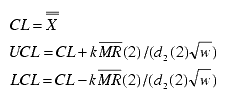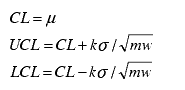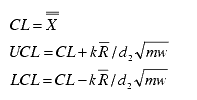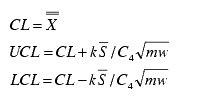# Moving XBar chart calculations

XBar-S chart control limits are calculated as follows:

 Control Limits calculated from data Control Limits calculated from standard (mean ( µ ) and/or standard deviation (s ) are given) If subgroup size = 1If subgroup size >1 and <=25same as subgroup size = 1 If subgroup size >25same as subgroup size = 1

NOTE: For the Moving XBar chart, control limit values are variable for the first set of subgroups (the number is equal to the moving length). After the first set of subgroups the control limit values are constant. If control limits are specified for the Moving XBar chart, then the specified limits are constant and the system will calculate the initial variable control limit.

NOTE: For ith subgroups, where i<w, use i instead of w in the above formulae.

NOTE: If subgroup size is variable, m is replaced with mi for ith subgroup limits. When subgroup size is variable, the control limit values will not be the same; therefore, the chart may display `city skyline' type control lines.

## Data Plot Items

• Moving Average

• Lower Control Limit (LCL) (optional)

• Upper Control Limit (UCL) (optional)

• Warning lines (optional)

## Descriptive Statistics

• Lower Control Limit (LCL)

• Upper Control Limit (UCL)

• Center Line (CL)